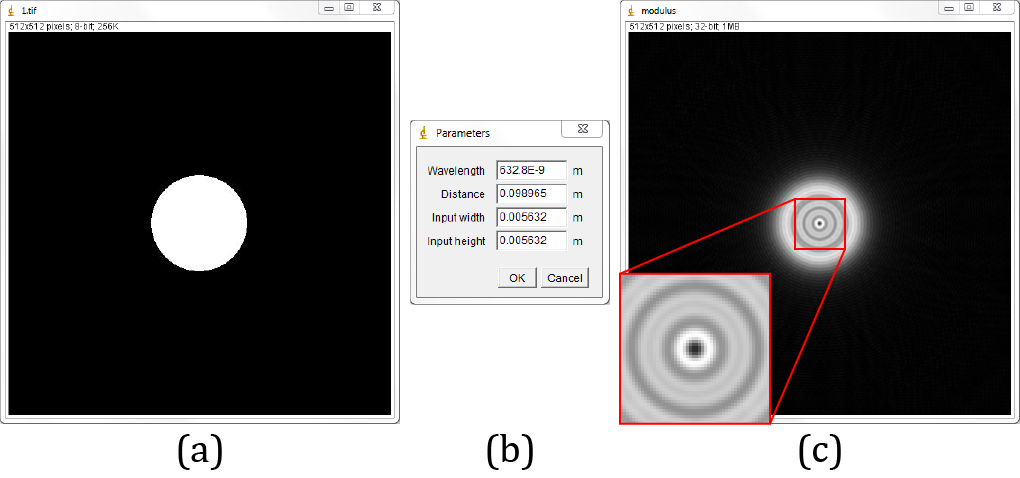JDiffraction is a numerical wave propagation library for Java. Includes angular spectrum, Fresnel-Fourier and Fresnel-Bluestein methods. Aditionally it includes an utilities class, designed to work with the complex arrays required by the library.

The library supports calculation on CPU and GPU, the latter is done using JCuda. To use the GPGPU versions of the library JCuda 0.7.5 jars must be in the java path, CUDA 7.5 and a CUDA capable GPU must be installed on the PC.

### Examples

An example of usage of JDiffraction on a simple ImageJ's plugin. Diffraction of a circular aperture using the angular spectrum method. The parameters are meant to produce an even number of Fresnel zones on the diffraction pattern. The example can be downloaded as a `.java` file here.(a) Circular aperture. (b) GenericDialog for parameters. (c) Modulus of the propagated field.

#### Code:

```import ij.*;
import ij.gui.GenericDialog;
import ij.process.*;
import ij.plugin.filter.*;
import unal.od.jdiffraction.cpu.FloatAngularSpectrum;
import unal.od.jdiffraction.cpu.utils.ArrayUtils;

public class JDiffraction_Example implements PlugInFilter {

ImagePlus imp;

@Override
public int setup(String arg, ImagePlus imp) {
this.imp = imp;
return DOES_ALL;
}

@Override
public void run(ImageProcessor ip) {
//gets the image data and creates the complex array
int M = ip.getWidth();
int N = ip.getHeight();

float[][] image = ip.getFloatArray();
float[][] field = ArrayUtils.complexAmplitude2(image, null);

//unlocks the image because it's no longer needed
imp.unlock();

//Creates a GenericDialog object to ask the user for propagation
//parameters
GenericDialog gd = new GenericDialog("Parameters");
gd.addNumericField("Input width", 5E-3, 4, 10, "m");
gd.addNumericField("Input height", 5E-3, 4, 10, "m");
gd.showDialog();

if (gd.wasCanceled()){
return;
}

float wavelength = (float) gd.getNextNumber();
float distance = (float) gd.getNextNumber();
float inputWidth = (float) gd.getNextNumber();
float inputHeight = (float) gd.getNextNumber();

//Creates the AngularSpectrum object and diffracts the input field
FloatAngularSpectrum as = new FloatAngularSpectrum(M, N, wavelength,
distance, inputWidth / M, inputHeight / N);
as.diffract(field);

//Calculates the modulus of the output field and shows it
float[][] modulus = ArrayUtils.modulus(field);

ImageProcessor ipModulus = new FloatProcessor(modulus);
ImagePlus impModulus = new ImagePlus("modulus", ipModulus);
impModulus.show();
}
}
```

### Benchmark

The library was benchmarked in its single precision versions, using a desktop PC with Intel Core i7 2600 (3.40 GHz), 8 GB of RAM memory and a NVIDIA GeForce GTX 580. The average times of execution are given in ms.

#### CPU times with and without constructor

Image Size (Pixels) Angular spectrum Fresnel - Fourier Fresnel - Bluestein
w/ const. w/o const. w/ const. w/o const. w/ const. w/o const.
512 x 512 53.2 5.4 67.9 4.1 88.5 7
1024 x 1024 215.6 24.8 270.3 16.2 357.8 30.4
2048 x 2048 874.9 111.6 1115.8 71.7 1486.3 135.7

#### GPU times with and without constructor

Image Size (Pixels) Angular spectrum Fresnel - Fourier Fresnel - Bluestein
w/ const. w/o const. w/ const. w/o const. w/ const. w/o const.
512 x 512 3.4 2.5 3 2.1 4.2 3.4
1024 x 1024 5.9 4.8 4.8 4.1 8.1 7.2
2048 x 2048 15.5 15 14.1 13.5 22.4 21.9

### API

JDiffraction API can be found here.

### Credits

JDiffraction uses JTransforms FFT routines and JCuda Java bindings for NVIDIA CUDA.

### Citation

You can reference JDiffraction using:

1. P. Piedrahita-Quintero, C. Trujillo, and J. Garcia-Sucerquia, "JDiffraction: A GPGPU-accelerated JAVA library for numerical propagation of scalar wave fields," Comput. Phys. Commun. 214, (2017). doi: 10.1016/j.cpc.2016.12.016